Скачать презентацию Power Point Slides by Ron Cronovich CHAPTER 4

b49db1361d7fcfa934c4af3fda70f76a.ppt

• Количество слайдов: 69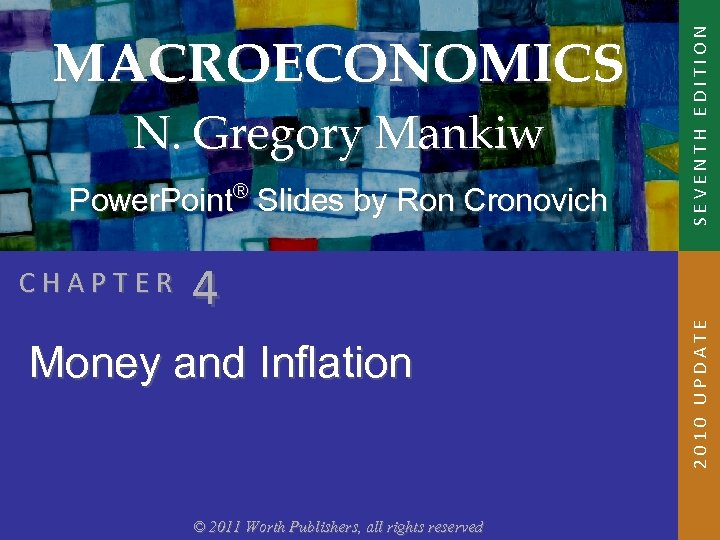Power. Point® Slides by Ron Cronovich CHAPTER 4 Money and Inflation © 2011 Worth Publishers, all rights reserved SEVENTH EDITION N. Gregory Mankiw 2010 UPDATE MACROECONOMICS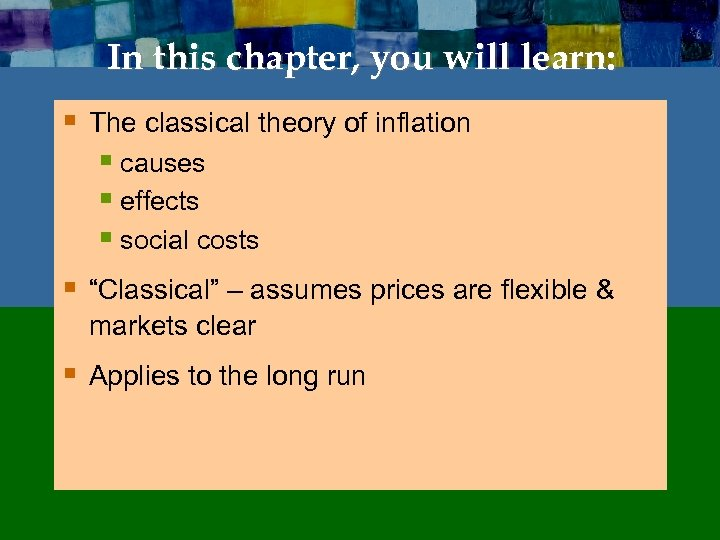In this chapter, you will learn: § The classical theory of inflation § causes § effects § social costs § “Classical” – assumes prices are flexible & markets clear § Applies to the long run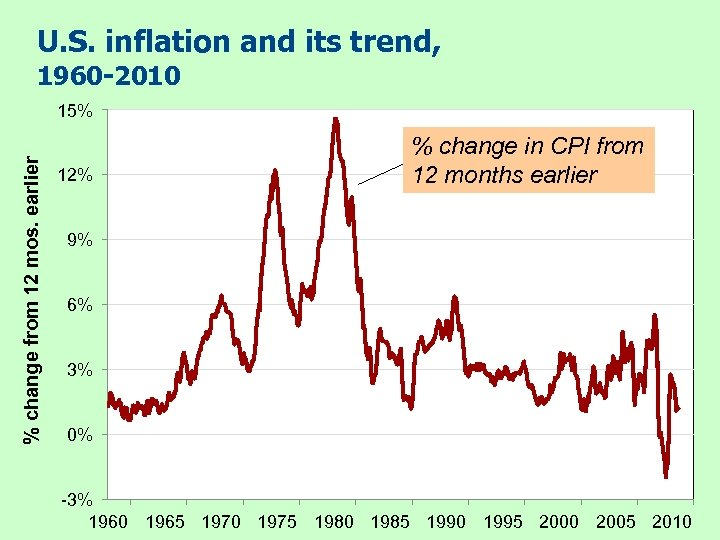U. S. inflation and its trend, 1960 -2010 % change from 12 mos. earlier 15% 12% % change in CPI from 12 months earlier 9% 6% 3% 0% -3% 1960 1965 1970 1975 1980 1985 1990 1995 2000 2005 2010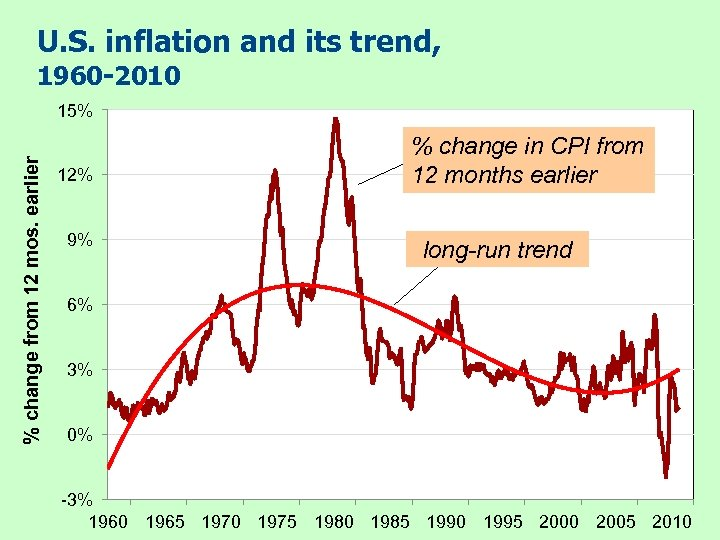U. S. inflation and its trend, 1960 -2010 % change from 12 mos. earlier 15% 12% 9% % change in CPI from 12 months earlier long-run trend 6% 3% 0% -3% 1960 1965 1970 1975 1980 1985 1990 1995 2000 2005 2010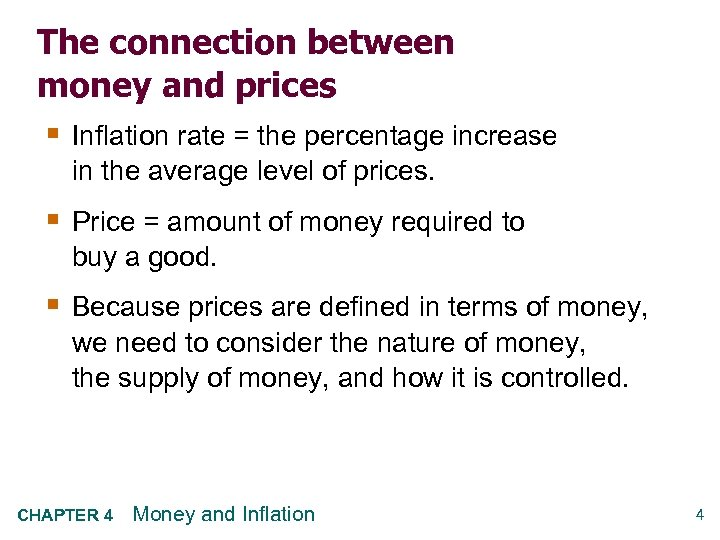The connection between money and prices § Inflation rate = the percentage increase in the average level of prices. § Price = amount of money required to buy a good. § Because prices are defined in terms of money, we need to consider the nature of money, the supply of money, and how it is controlled. CHAPTER 4 Money and Inflation 4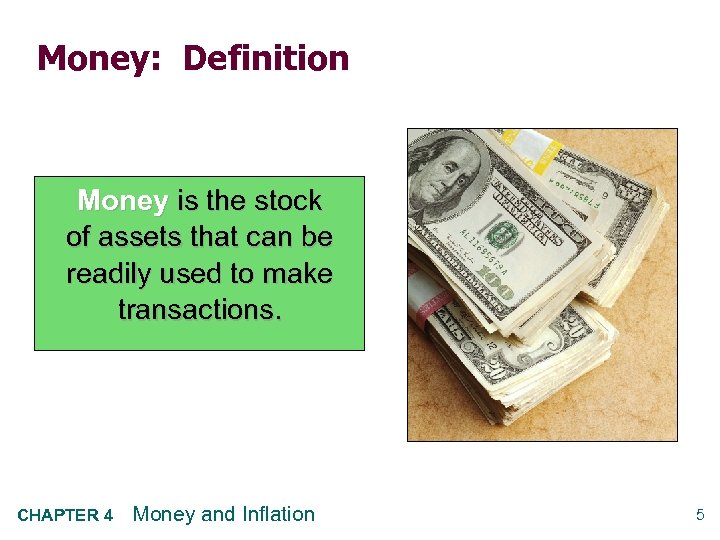Money: Definition Money is the stock of assets that can be readily used to make transactions. CHAPTER 4 Money and Inflation 5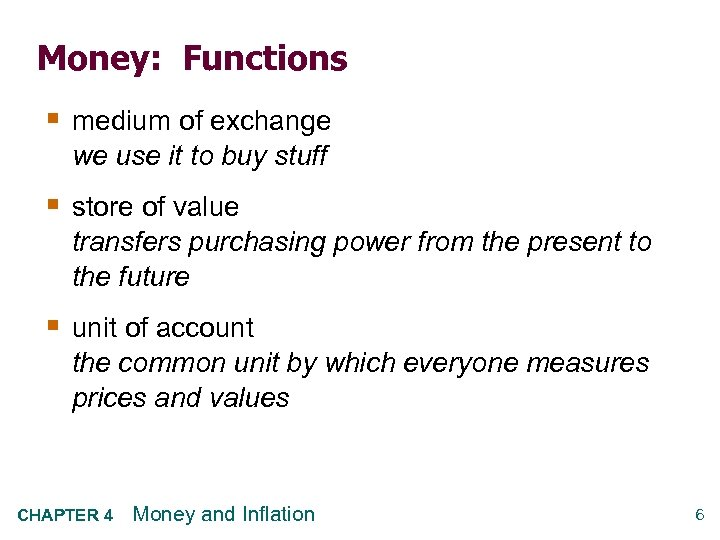Money: Functions § medium of exchange we use it to buy stuff § store of value transfers purchasing power from the present to the future § unit of account the common unit by which everyone measures prices and values CHAPTER 4 Money and Inflation 6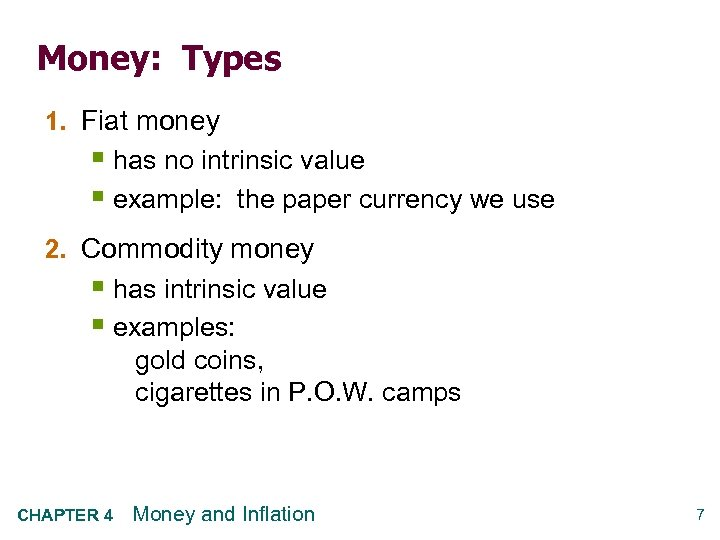Money: Types 1. Fiat money § has no intrinsic value § example: the paper currency we use 2. Commodity money § has intrinsic value § examples: gold coins, cigarettes in P. O. W. camps CHAPTER 4 Money and Inflation 7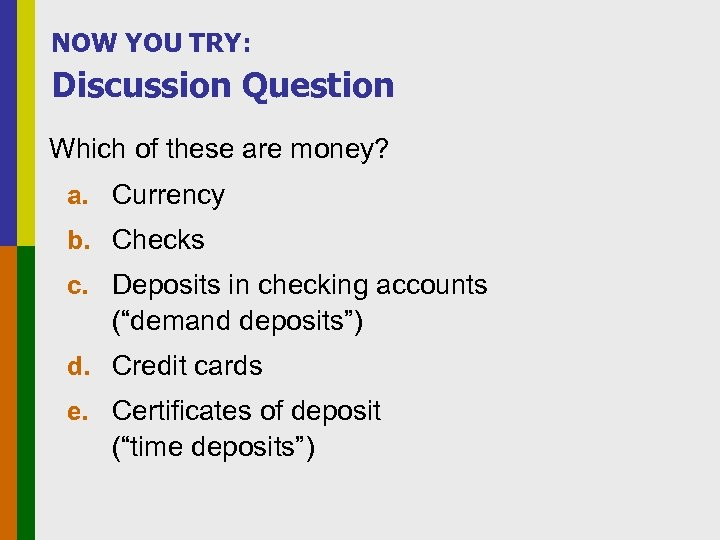NOW YOU TRY: Discussion Question Which of these are money? a. Currency b. Checks c. Deposits in checking accounts (“demand deposits”) d. Credit cards e. Certificates of deposit (“time deposits”)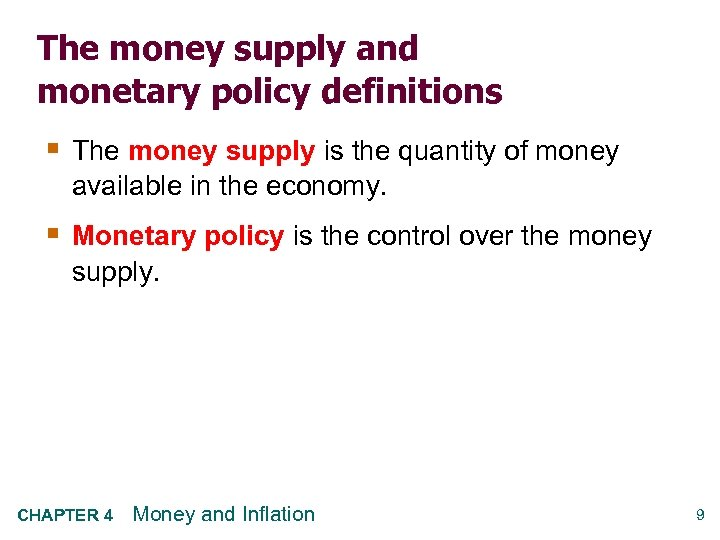The money supply and monetary policy definitions § The money supply is the quantity of money available in the economy. § Monetary policy is the control over the money supply. CHAPTER 4 Money and Inflation 9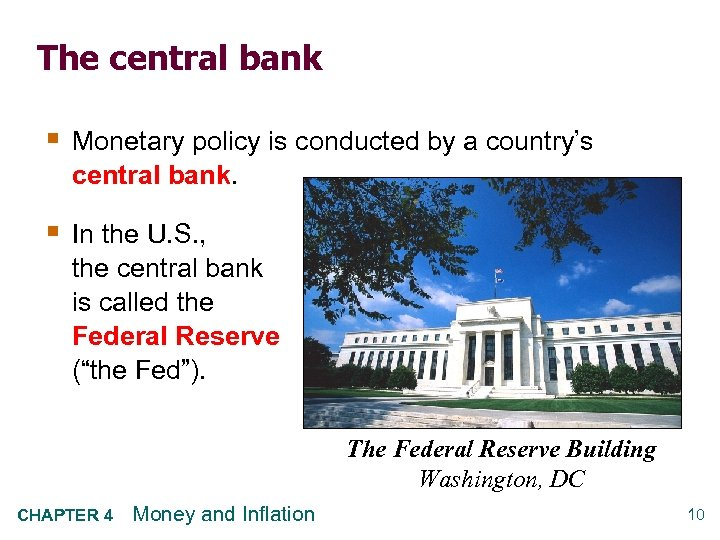The central bank § Monetary policy is conducted by a country’s central bank. § In the U. S. , the central bank is called the Federal Reserve (“the Fed”). The Federal Reserve Building Washington, DC CHAPTER 4 Money and Inflation 10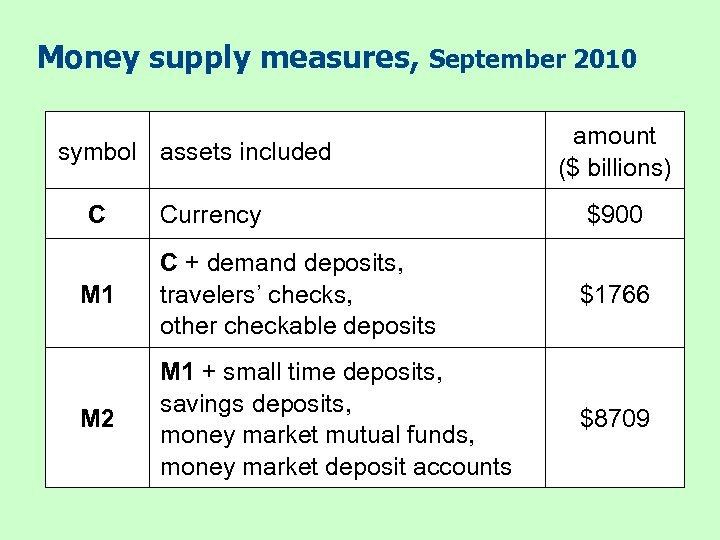Money supply measures, September 2010 symbol assets included C amount (\$ billions) Currency \$900 M 1 C + demand deposits, travelers’ checks, other checkable deposits \$1766 M 2 M 1 + small time deposits, savings deposits, money market mutual funds, money market deposit accounts \$8709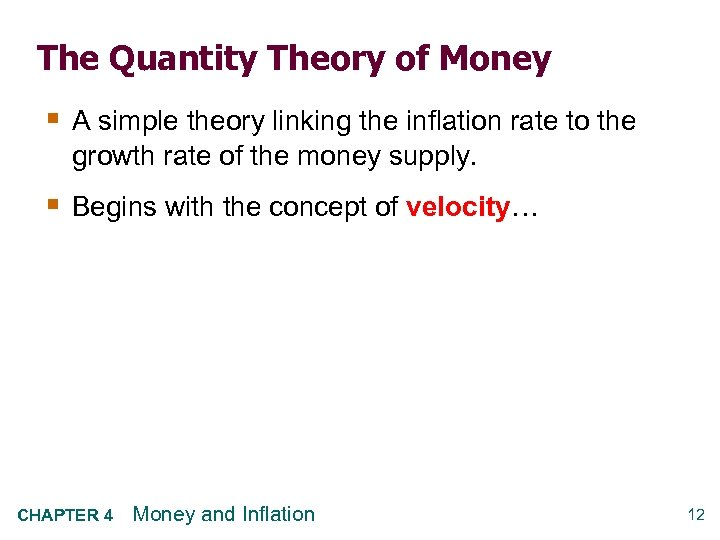The Quantity Theory of Money § A simple theory linking the inflation rate to the growth rate of the money supply. § Begins with the concept of velocity… CHAPTER 4 Money and Inflation 12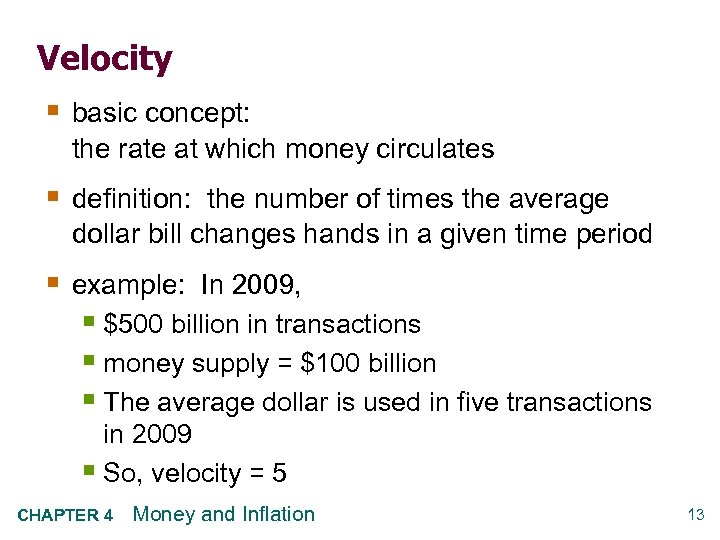Velocity § basic concept: the rate at which money circulates § definition: the number of times the average dollar bill changes hands in a given time period § example: In 2009, § \$500 billion in transactions § money supply = \$100 billion § The average dollar is used in five transactions in 2009 § So, velocity = 5 CHAPTER 4 Money and Inflation 13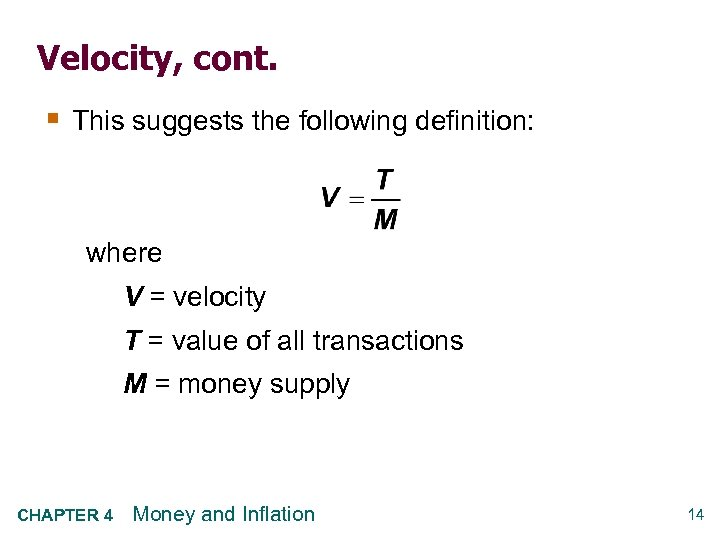Velocity, cont. § This suggests the following definition: where V = velocity T = value of all transactions M = money supply CHAPTER 4 Money and Inflation 14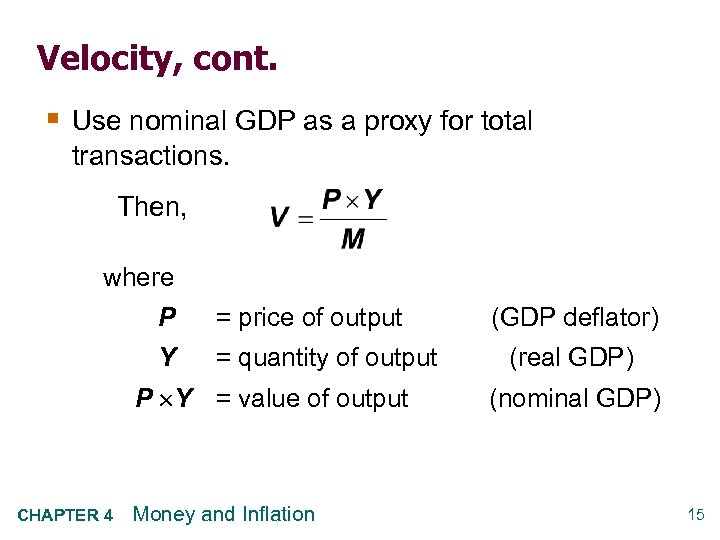Velocity, cont. § Use nominal GDP as a proxy for total transactions. Then, where P = price of output Y = quantity of output P Y = value of output CHAPTER 4 Money and Inflation (GDP deflator) (real GDP) (nominal GDP) 15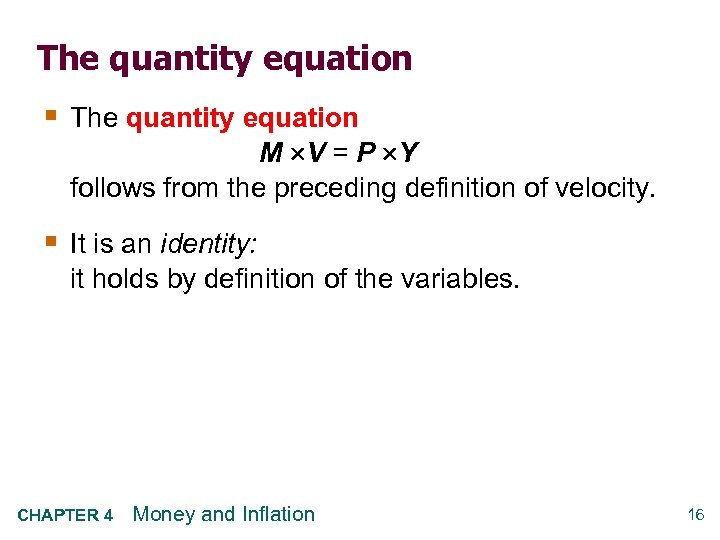The quantity equation § The quantity equation M V = P Y follows from the preceding definition of velocity. § It is an identity: it holds by definition of the variables. CHAPTER 4 Money and Inflation 16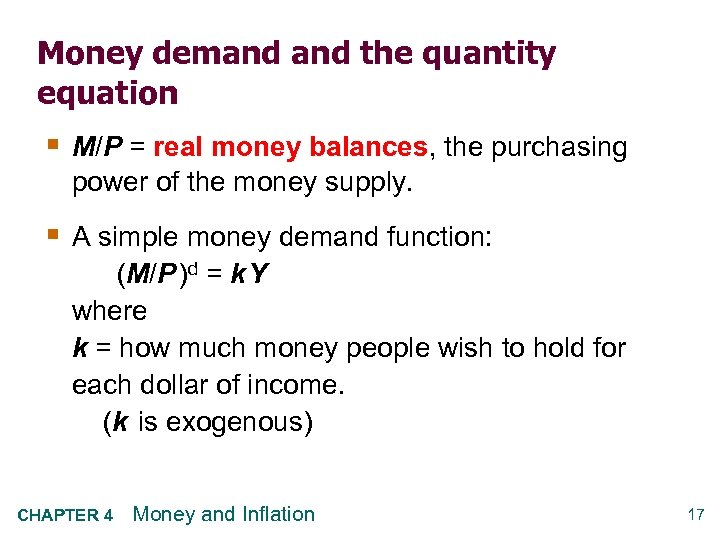Money demand the quantity equation § M/P = real money balances, the purchasing power of the money supply. § A simple money demand function: (M/P )d = k Y where k = how much money people wish to hold for each dollar of income. (k is exogenous) CHAPTER 4 Money and Inflation 17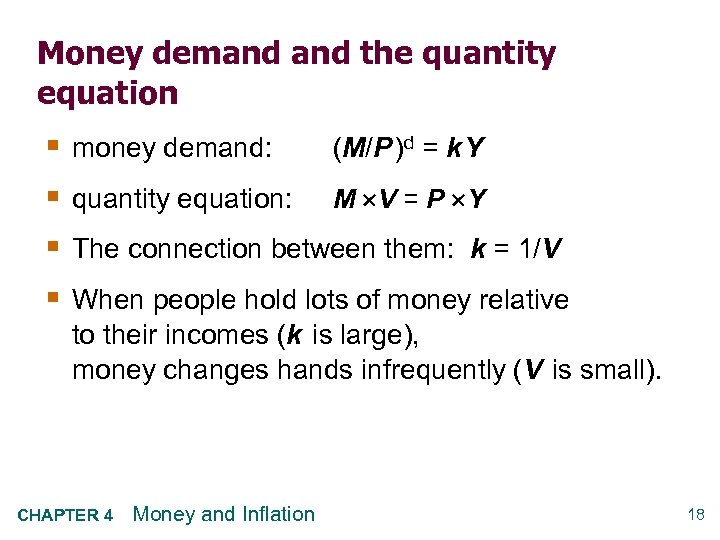Money demand the quantity equation § § money demand: (M/P )d = k Y quantity equation: M V = P Y The connection between them: k = 1/V When people hold lots of money relative to their incomes (k is large), money changes hands infrequently (V is small). CHAPTER 4 Money and Inflation 18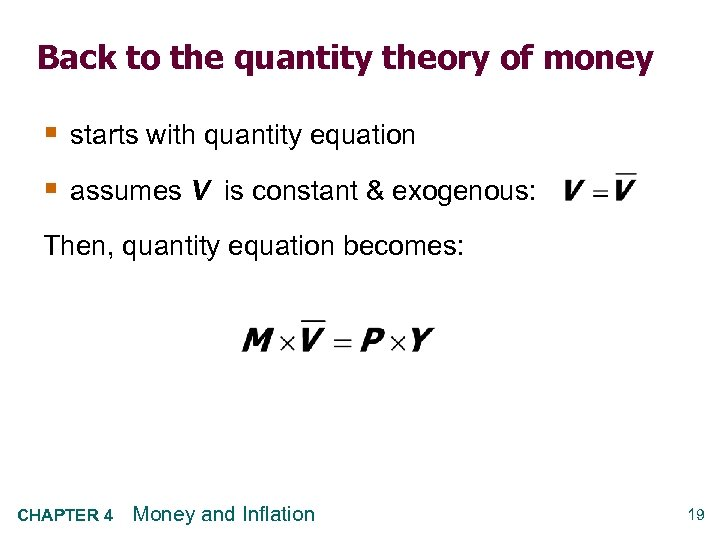Back to the quantity theory of money § starts with quantity equation § assumes V is constant & exogenous: Then, quantity equation becomes: CHAPTER 4 Money and Inflation 19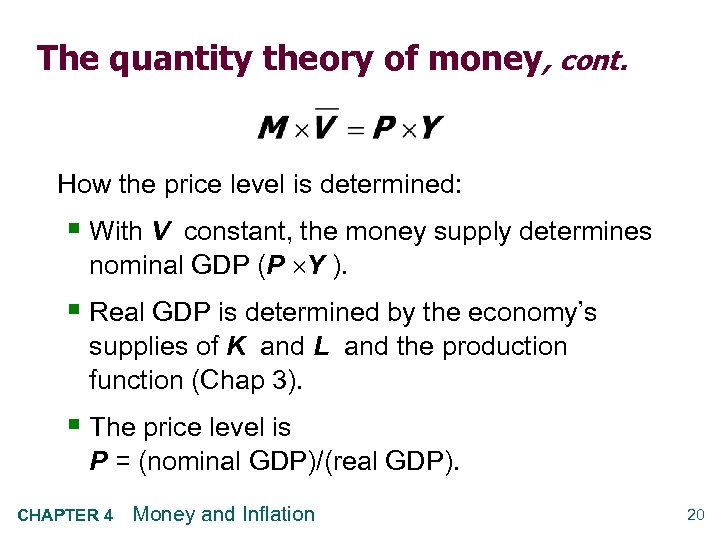The quantity theory of money, cont. How the price level is determined: § With V constant, the money supply determines nominal GDP (P Y ). § Real GDP is determined by the economy’s supplies of K and L and the production function (Chap 3). § The price level is P = (nominal GDP)/(real GDP). CHAPTER 4 Money and Inflation 20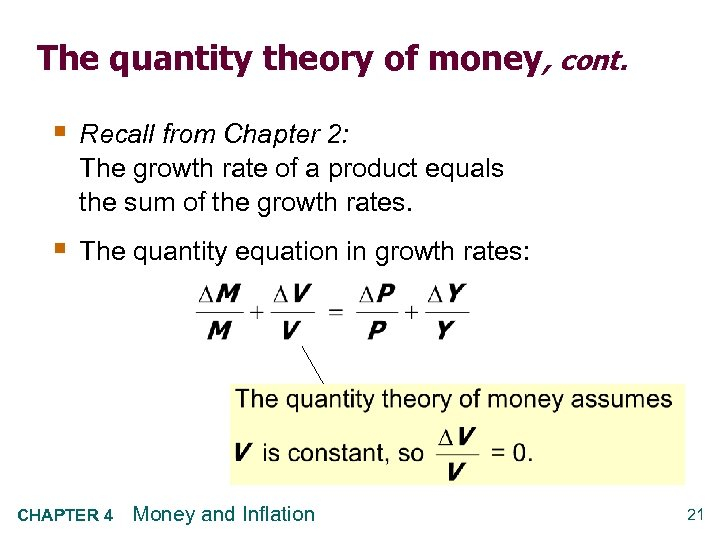The quantity theory of money, cont. § Recall from Chapter 2: The growth rate of a product equals the sum of the growth rates. § The quantity equation in growth rates: CHAPTER 4 Money and Inflation 21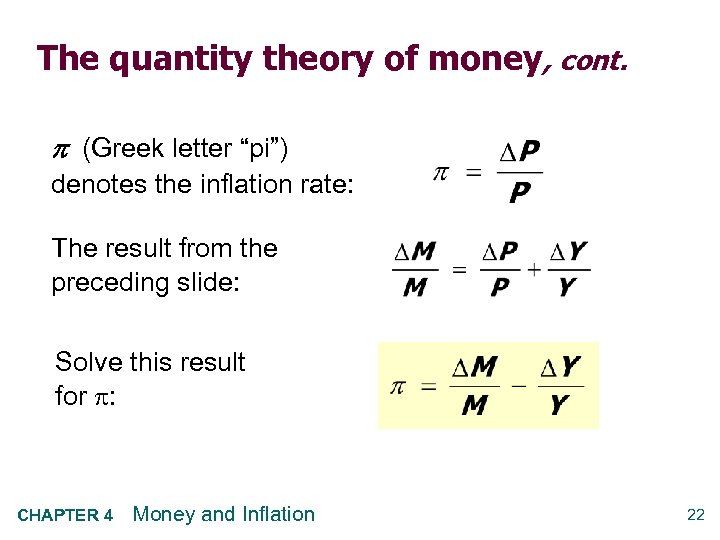The quantity theory of money, cont. (Greek letter “pi”) denotes the inflation rate: The result from the preceding slide: Solve this result for : CHAPTER 4 Money and Inflation 22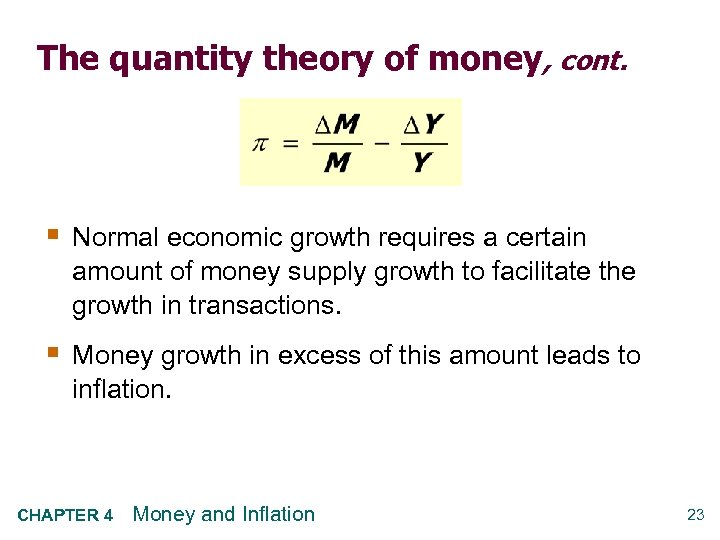The quantity theory of money, cont. § Normal economic growth requires a certain amount of money supply growth to facilitate the growth in transactions. § Money growth in excess of this amount leads to inflation. CHAPTER 4 Money and Inflation 23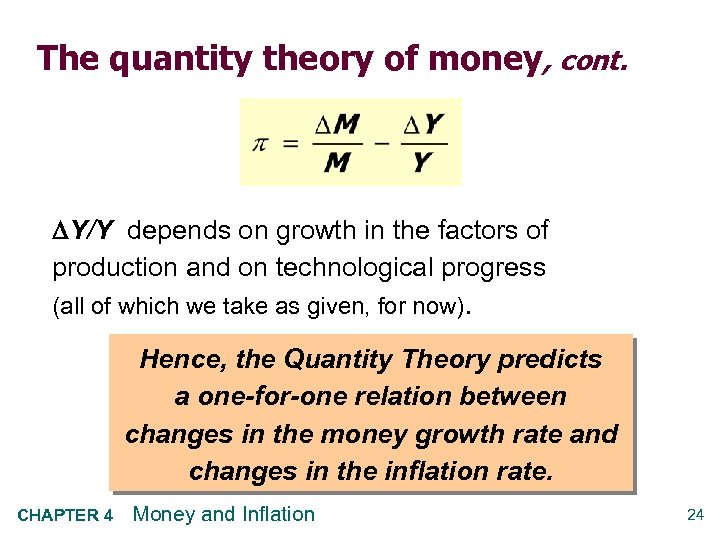The quantity theory of money, cont. Y/Y depends on growth in the factors of production and on technological progress (all of which we take as given, for now). Hence, the Quantity Theory predicts a one-for-one relation between changes in the money growth rate and changes in the inflation rate. CHAPTER 4 Money and Inflation 24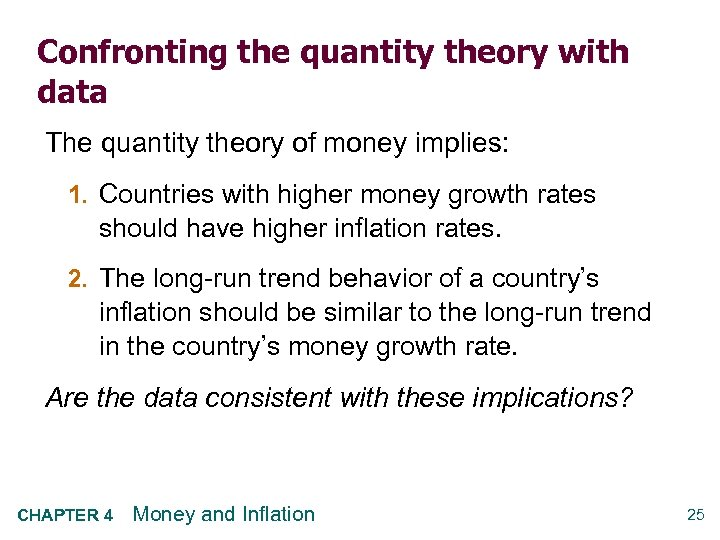Confronting the quantity theory with data The quantity theory of money implies: 1. Countries with higher money growth rates should have higher inflation rates. 2. The long-run trend behavior of a country’s inflation should be similar to the long-run trend in the country’s money growth rate. Are the data consistent with these implications? CHAPTER 4 Money and Inflation 25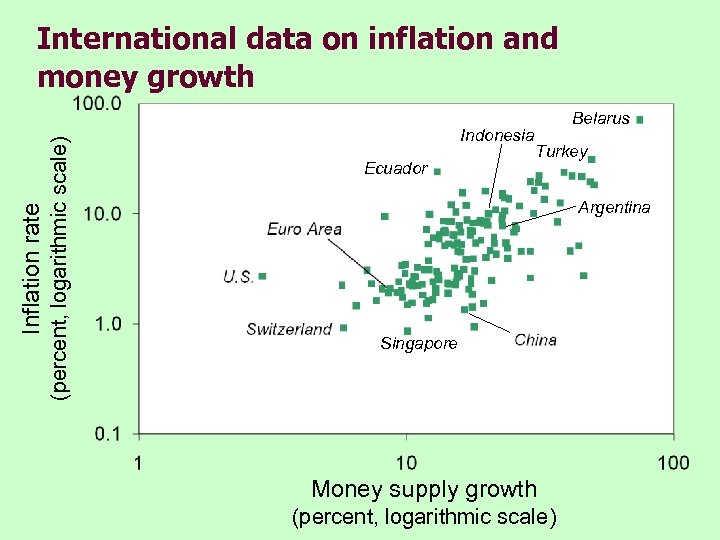(percent, logarithmic scale) Inflation rate International data on inflation and money growth Indonesia Ecuador Belarus Turkey Argentina Singapore Money supply growth (percent, logarithmic scale)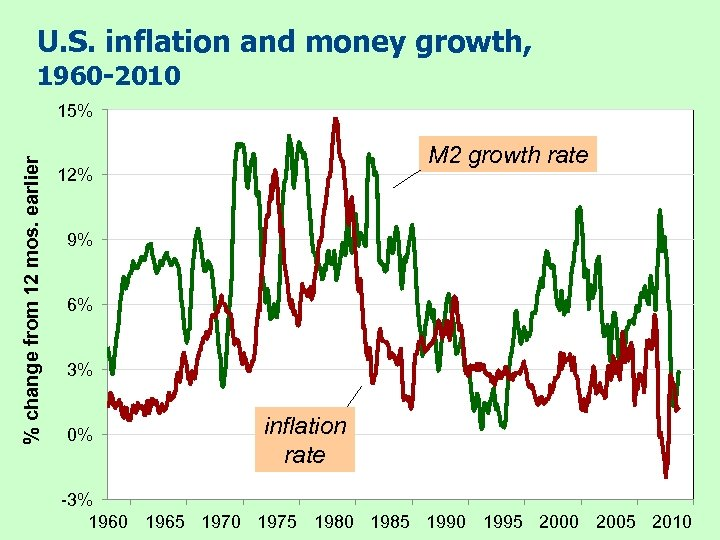U. S. inflation and money growth, 1960 -2010 % change from 12 mos. earlier 15% M 2 growth rate 12% 9% 6% 3% 0% inflation rate -3% 1960 1965 1970 1975 1980 1985 1990 1995 2000 2005 2010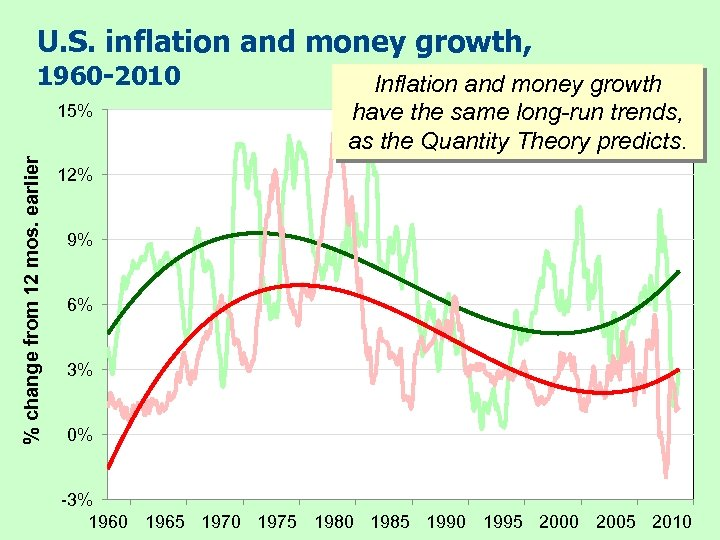U. S. inflation and money growth, 1960 -2010 % change from 12 mos. earlier 15% Inflation and money growth have the same long-run trends, as the Quantity Theory predicts. 12% 9% 6% 3% 0% -3% 1960 1965 1970 1975 1980 1985 1990 1995 2000 2005 2010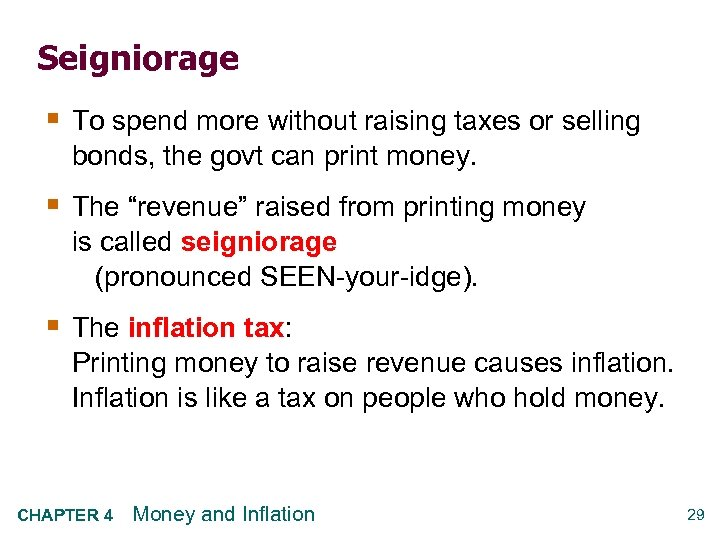Seigniorage § To spend more without raising taxes or selling bonds, the govt can print money. § The “revenue” raised from printing money is called seigniorage (pronounced SEEN-your-idge). § The inflation tax: Printing money to raise revenue causes inflation. Inflation is like a tax on people who hold money. CHAPTER 4 Money and Inflation 29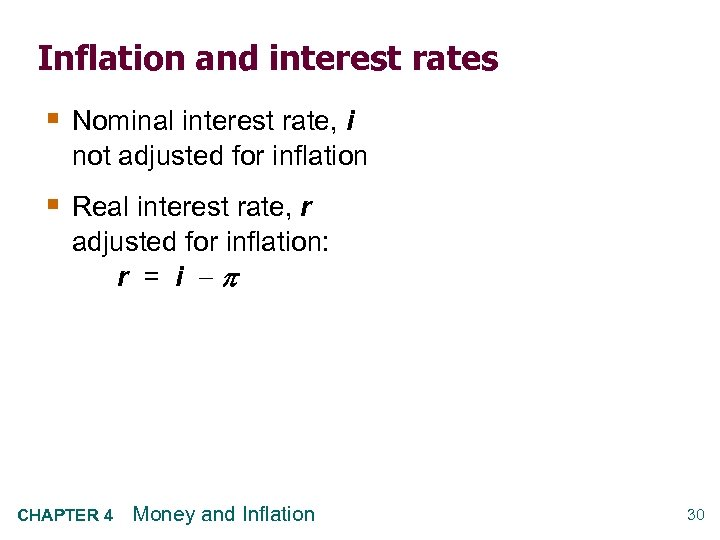Inflation and interest rates § Nominal interest rate, i not adjusted for inflation § Real interest rate, r adjusted for inflation: r = i CHAPTER 4 Money and Inflation 30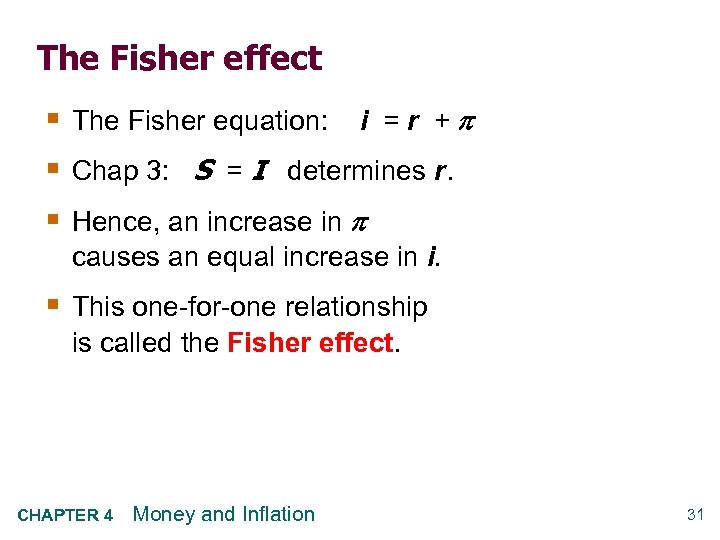The Fisher effect § The Fisher equation: i = r + § Chap 3: S = I determines r. § Hence, an increase in causes an equal increase in i. § This one-for-one relationship is called the Fisher effect. CHAPTER 4 Money and Inflation 31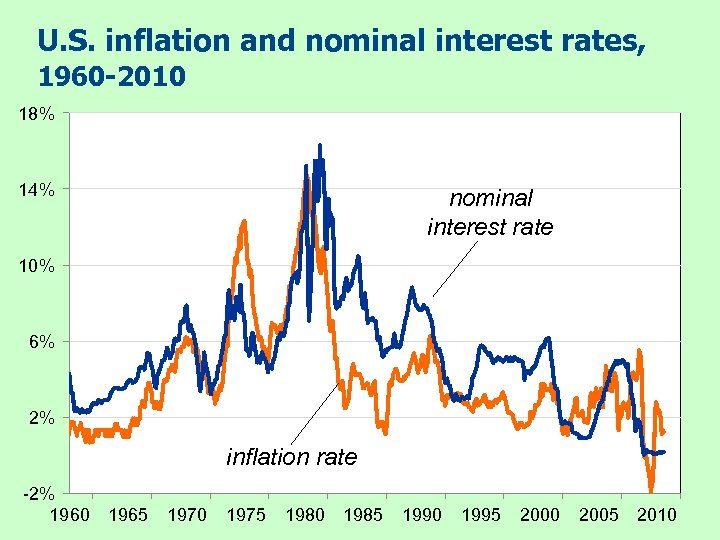U. S. inflation and nominal interest rates, 1960 -2010 18% 14% nominal interest rate 10% 6% 2% inflation rate -2% 1960 1965 1970 1975 1980 1985 1990 1995 2000 2005 2010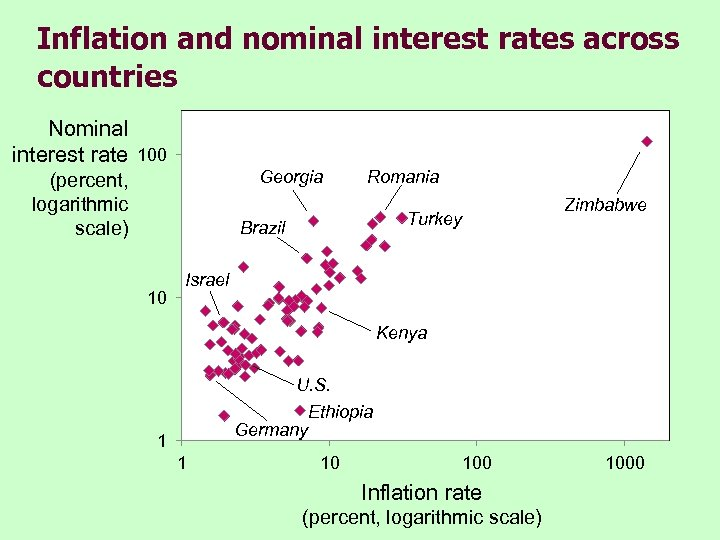Inflation and nominal interest rates across countries Nominal interest rate 100 Georgia (percent, logarithmic scale) Romania Turkey Brazil 10 Zimbabwe Israel Kenya U. S. Ethiopia Germany 1 1 10 100 Inflation rate (percent, logarithmic scale) 1000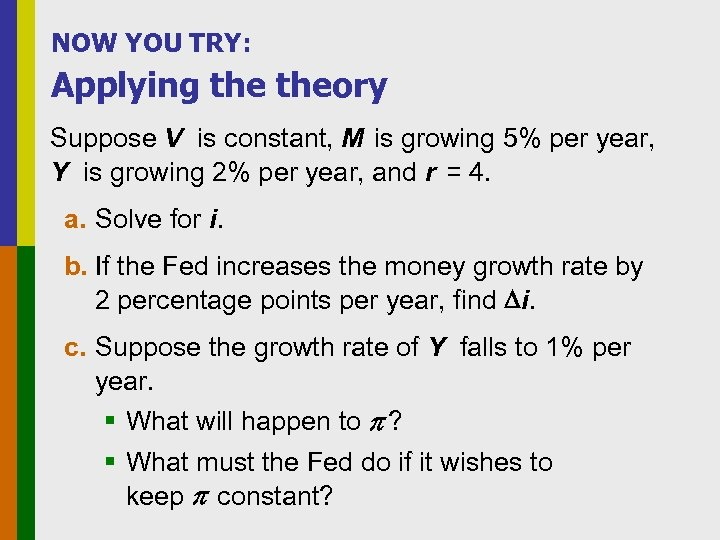NOW YOU TRY: Applying theory Suppose V is constant, M is growing 5% per year, Y is growing 2% per year, and r = 4. a. Solve for i. b. If the Fed increases the money growth rate by 2 percentage points per year, find i. c. Suppose the growth rate of Y falls to 1% per year. § What will happen to ? § What must the Fed do if it wishes to keep constant?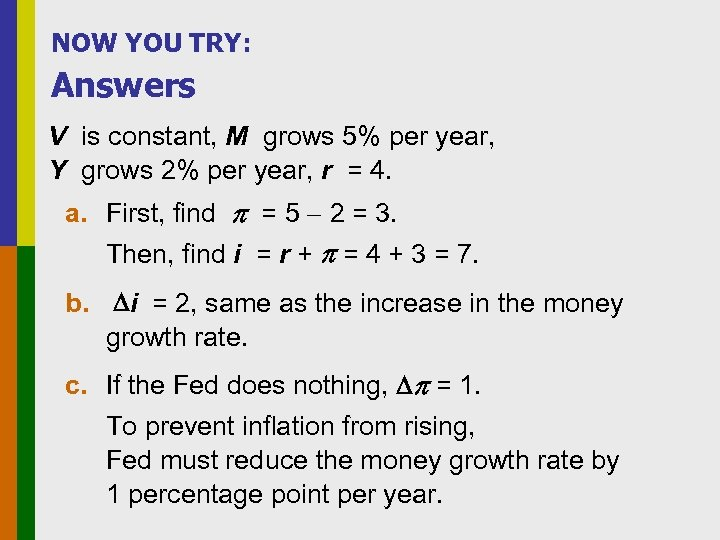NOW YOU TRY: Answers V is constant, M grows 5% per year, Y grows 2% per year, r = 4. a. First, find = 5 2 = 3. Then, find i = r + = 4 + 3 = 7. b. i = 2, same as the increase in the money growth rate. c. If the Fed does nothing, = 1. To prevent inflation from rising, Fed must reduce the money growth rate by 1 percentage point per year.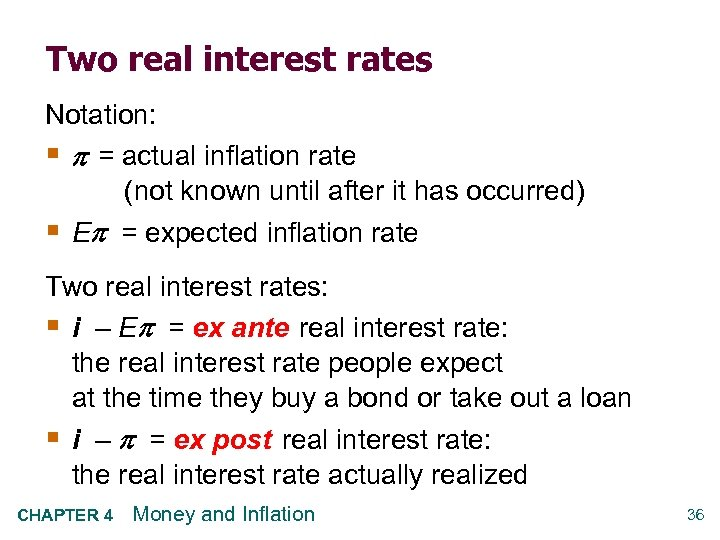Two real interest rates Notation: § = actual inflation rate (not known until after it has occurred) § E = expected inflation rate Two real interest rates: § i – E = ex ante real interest rate: the real interest rate people expect at the time they buy a bond or take out a loan § i – = ex post real interest rate: the real interest rate actually realized CHAPTER 4 Money and Inflation 36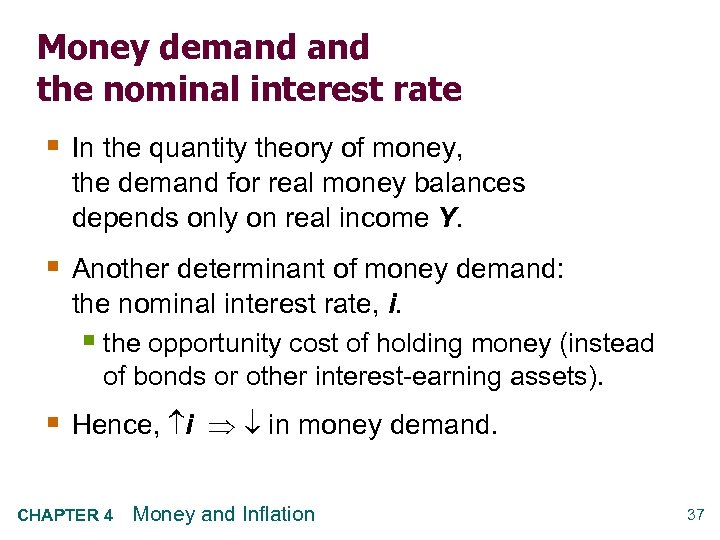Money demand the nominal interest rate § In the quantity theory of money, the demand for real money balances depends only on real income Y. § Another determinant of money demand: the nominal interest rate, i. § the opportunity cost of holding money (instead of bonds or other interest-earning assets). § Hence, i in money demand. CHAPTER 4 Money and Inflation 37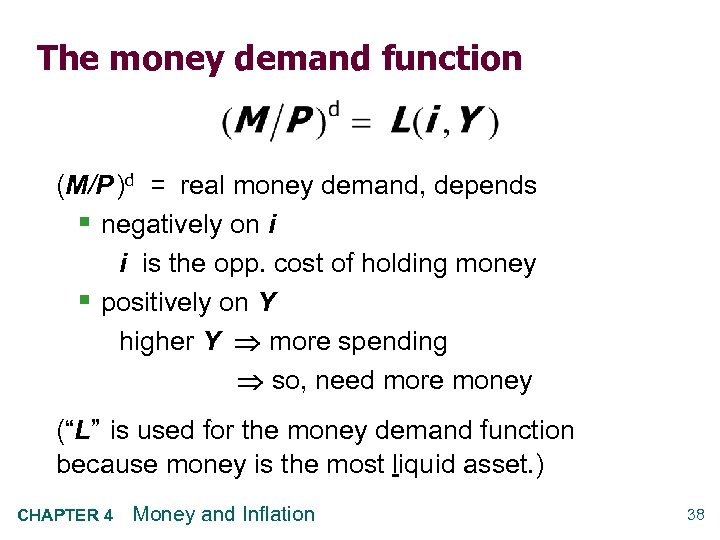The money demand function (M/P )d = real money demand, depends § negatively on i i is the opp. cost of holding money § positively on Y higher Y more spending so, need more money (“L” is used for the money demand function because money is the most liquid asset. ) CHAPTER 4 Money and Inflation 38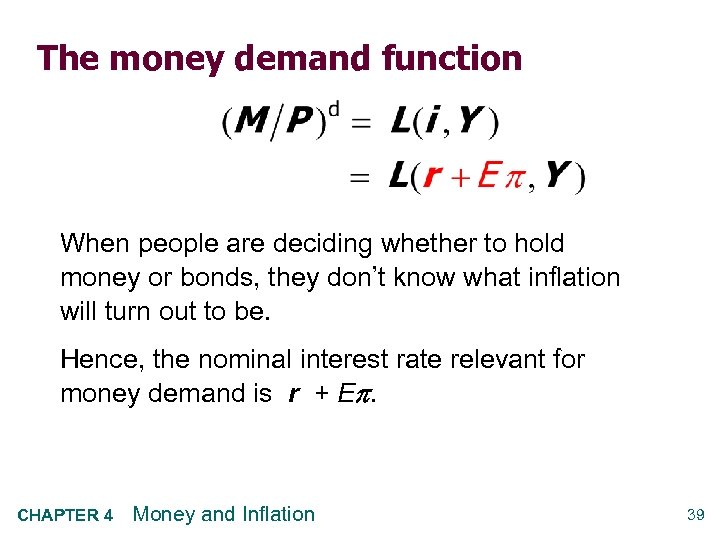The money demand function When people are deciding whether to hold money or bonds, they don’t know what inflation will turn out to be. Hence, the nominal interest rate relevant for money demand is r + E. CHAPTER 4 Money and Inflation 39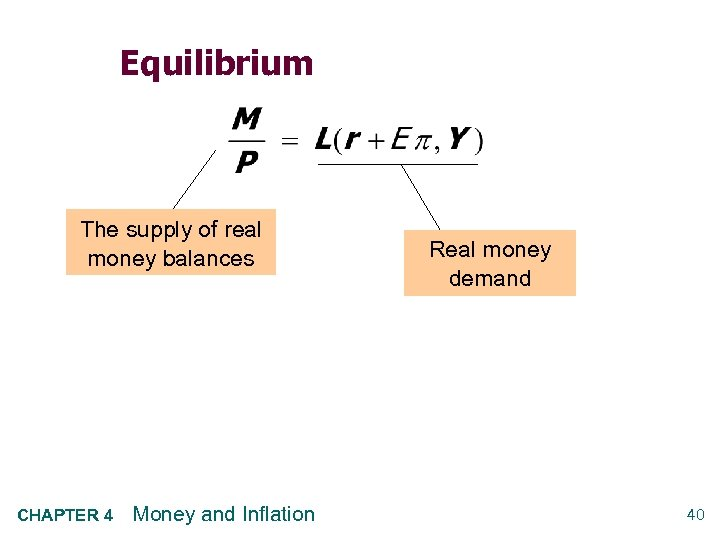Equilibrium The supply of real money balances CHAPTER 4 Money and Inflation Real money demand 40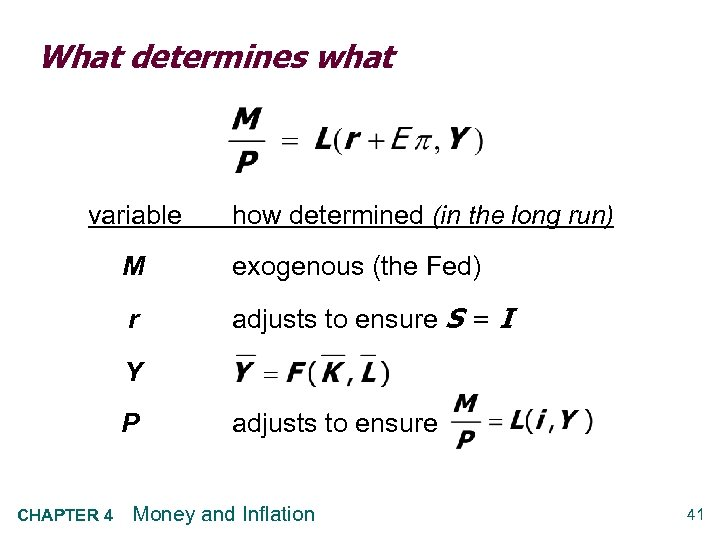What determines what variable how determined (in the long run) M exogenous (the Fed) r adjusts to ensure S = I Y P CHAPTER 4 adjusts to ensure Money and Inflation 41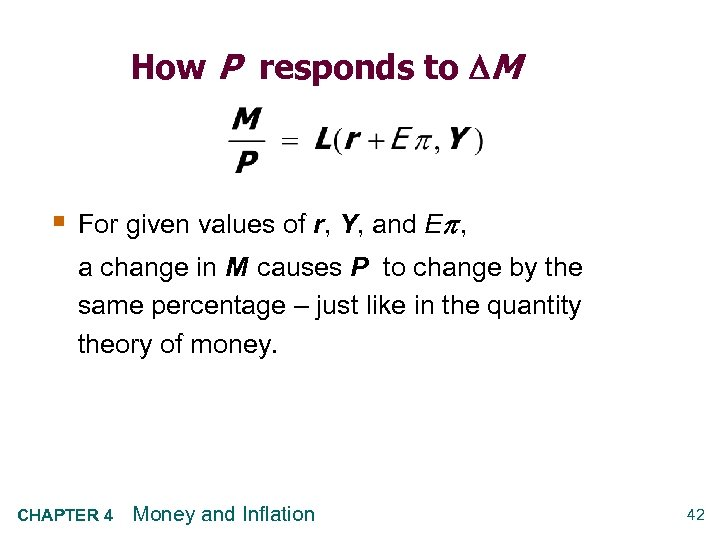How P responds to M § For given values of r, Y, and E , a change in M causes P to change by the same percentage – just like in the quantity theory of money. CHAPTER 4 Money and Inflation 42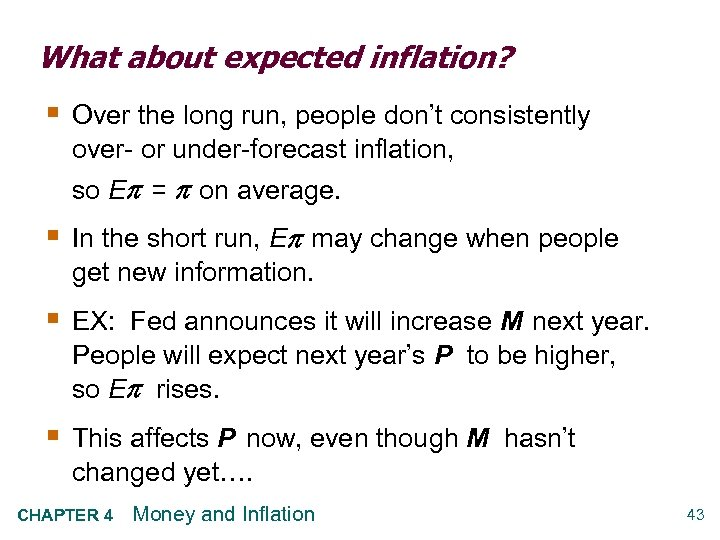What about expected inflation? § Over the long run, people don’t consistently over- or under-forecast inflation, so E = on average. § In the short run, E may change when people get new information. § EX: Fed announces it will increase M next year. People will expect next year’s P to be higher, so E rises. § This affects P now, even though M hasn’t changed yet…. CHAPTER 4 Money and Inflation 43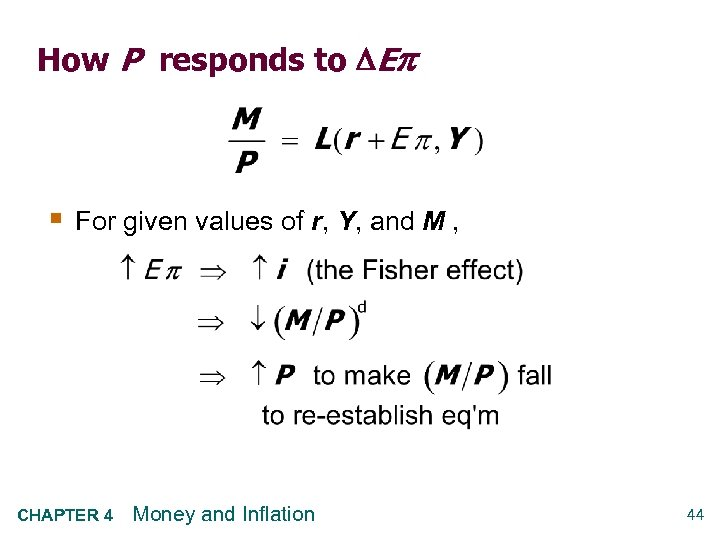How P responds to E § For given values of r, Y, and M , CHAPTER 4 Money and Inflation 44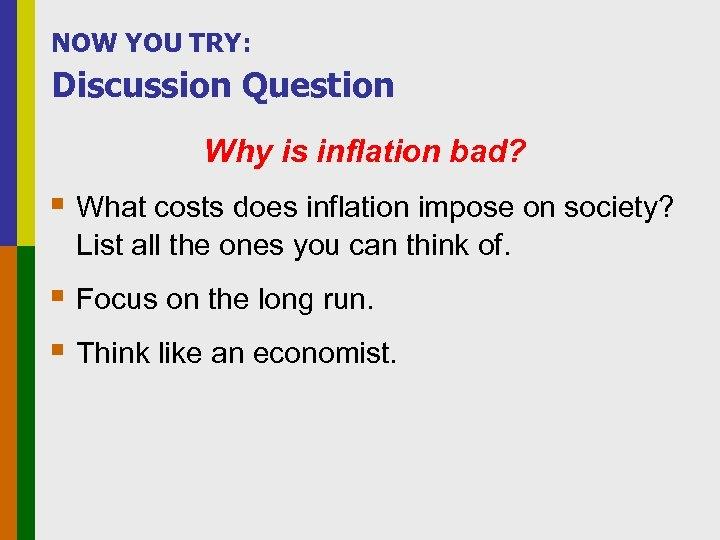NOW YOU TRY: Discussion Question Why is inflation bad? § What costs does inflation impose on society? List all the ones you can think of. § Focus on the long run. § Think like an economist.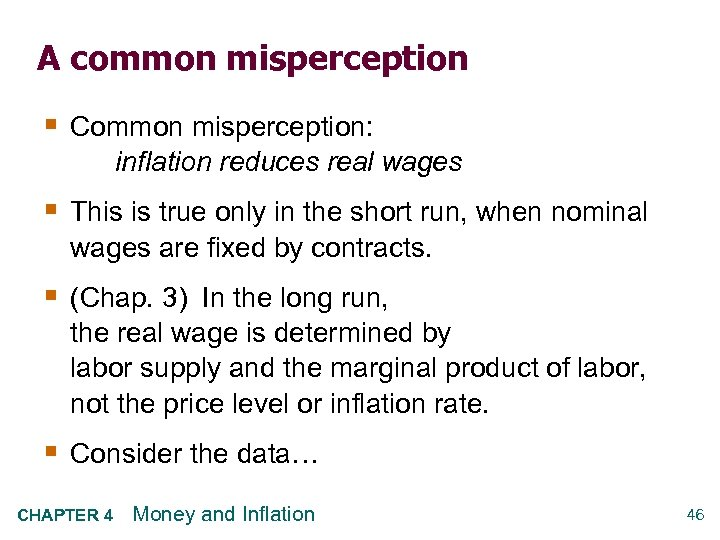A common misperception § Common misperception: inflation reduces real wages § This is true only in the short run, when nominal wages are fixed by contracts. § (Chap. 3) In the long run, the real wage is determined by labor supply and the marginal product of labor, not the price level or inflation rate. § Consider the data… CHAPTER 4 Money and Inflation 46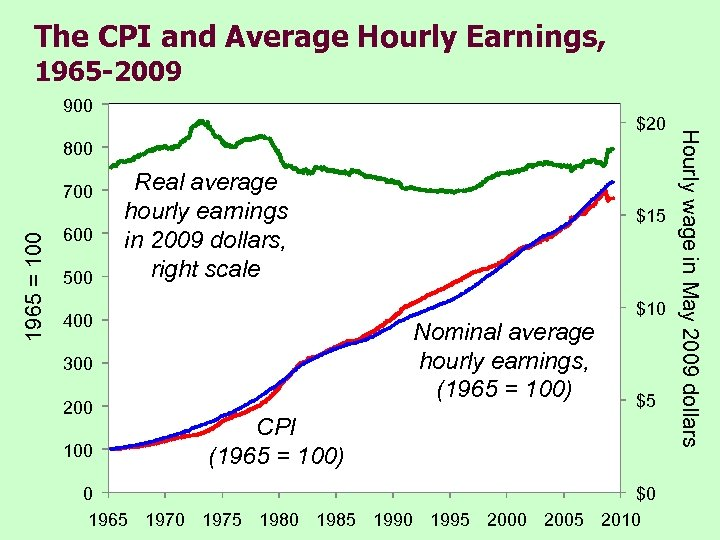The CPI and Average Hourly Earnings, 1965 -2009 900 800 1965 = 100 700 600 500 Real average hourly earnings in 2009 dollars, right scale 400 Nominal average hourly earnings, (1965 = 100) 300 200 100 \$15 \$10 \$5 CPI (1965 = 100) 0 \$0 1965 1970 1975 1980 1985 1990 1995 2000 2005 2010 Hourly wage in May 2009 dollars \$20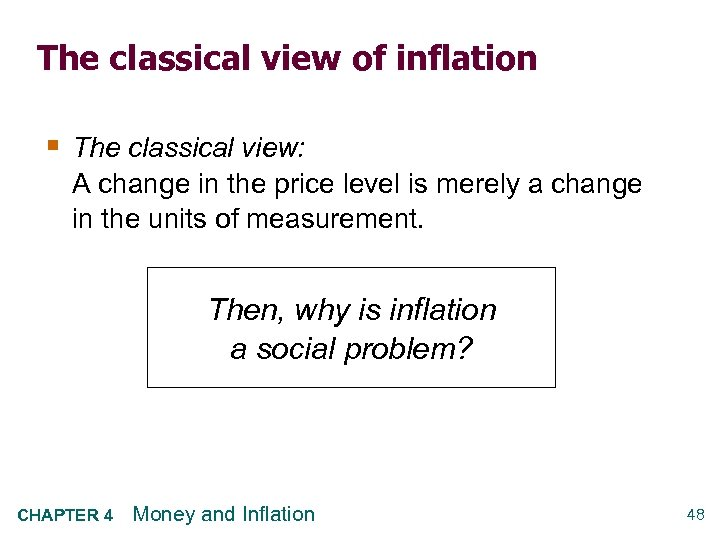The classical view of inflation § The classical view: A change in the price level is merely a change in the units of measurement. Then, why is inflation a social problem? CHAPTER 4 Money and Inflation 48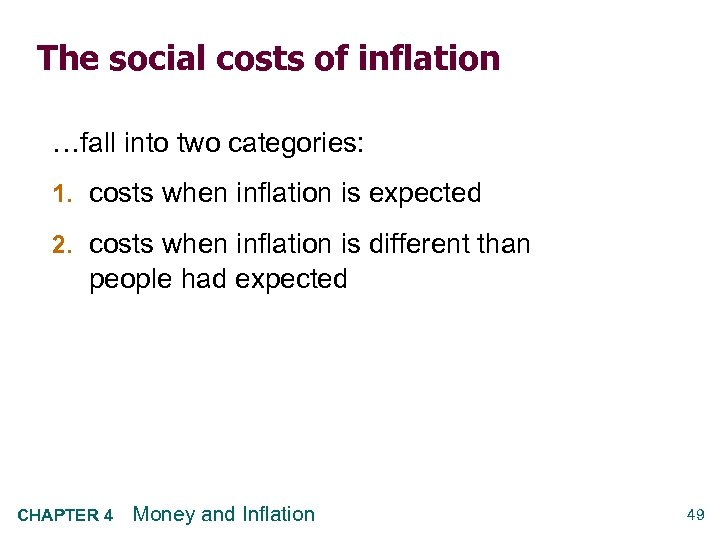The social costs of inflation …fall into two categories: 1. costs when inflation is expected 2. costs when inflation is different than people had expected CHAPTER 4 Money and Inflation 49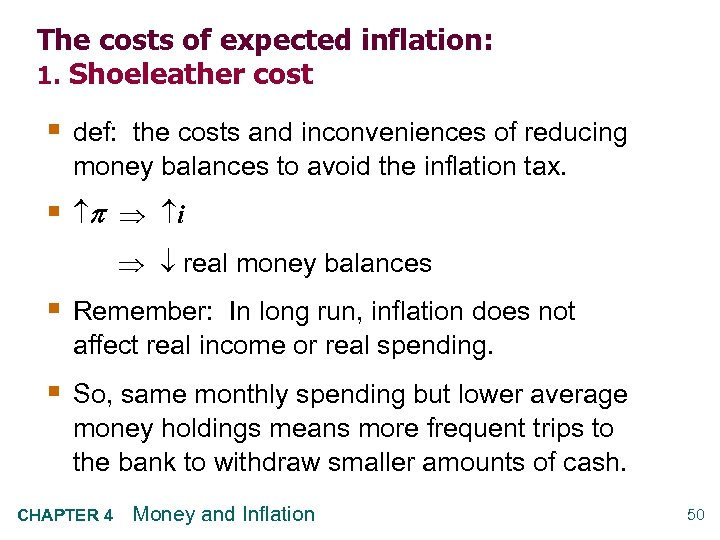The costs of expected inflation: 1. Shoeleather cost § def: the costs and inconveniences of reducing money balances to avoid the inflation tax. § i real money balances § Remember: In long run, inflation does not affect real income or real spending. § So, same monthly spending but lower average money holdings means more frequent trips to the bank to withdraw smaller amounts of cash. CHAPTER 4 Money and Inflation 50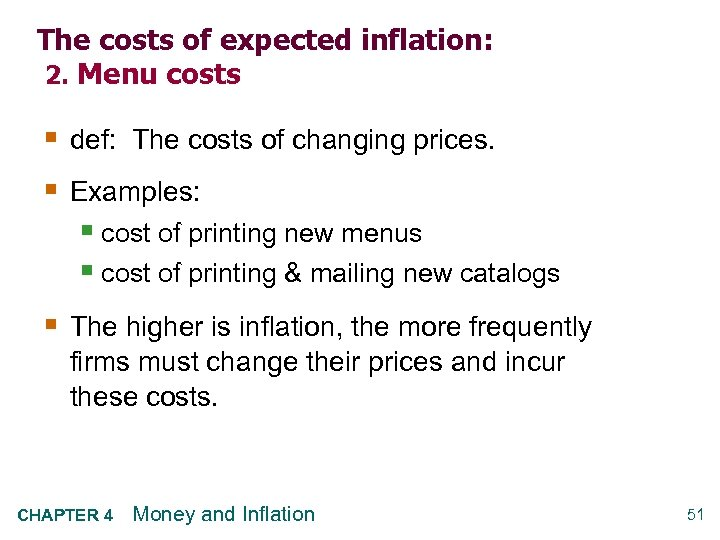The costs of expected inflation: 2. Menu costs § def: The costs of changing prices. § Examples: § cost of printing new menus § cost of printing & mailing new catalogs § The higher is inflation, the more frequently firms must change their prices and incur these costs. CHAPTER 4 Money and Inflation 51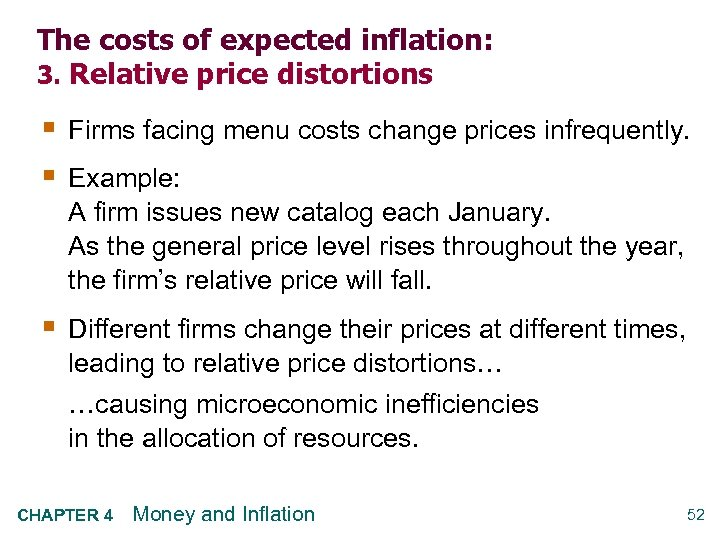The costs of expected inflation: 3. Relative price distortions § Firms facing menu costs change prices infrequently. § Example: A firm issues new catalog each January. As the general price level rises throughout the year, the firm’s relative price will fall. § Different firms change their prices at different times, leading to relative price distortions… …causing microeconomic inefficiencies in the allocation of resources. CHAPTER 4 Money and Inflation 52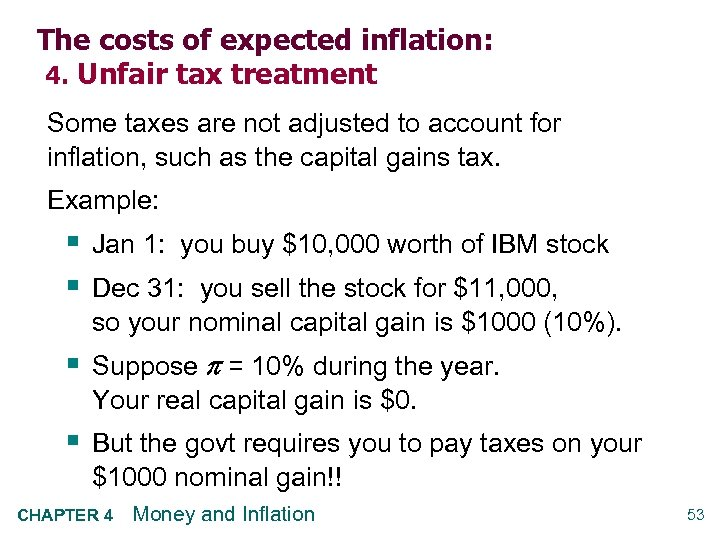The costs of expected inflation: 4. Unfair tax treatment Some taxes are not adjusted to account for inflation, such as the capital gains tax. Example: § Jan 1: you buy \$10, 000 worth of IBM stock § Dec 31: you sell the stock for \$11, 000, so your nominal capital gain is \$1000 (10%). § Suppose = 10% during the year. Your real capital gain is \$0. § But the govt requires you to pay taxes on your \$1000 nominal gain!! CHAPTER 4 Money and Inflation 53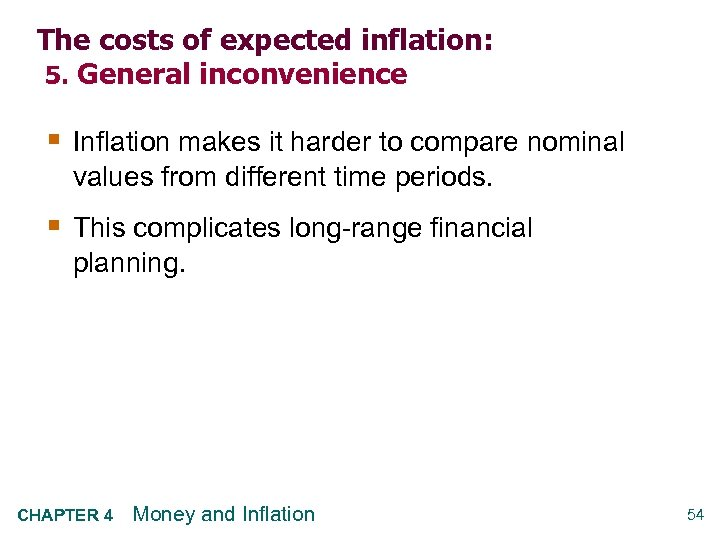The costs of expected inflation: 5. General inconvenience § Inflation makes it harder to compare nominal values from different time periods. § This complicates long-range financial planning. CHAPTER 4 Money and Inflation 54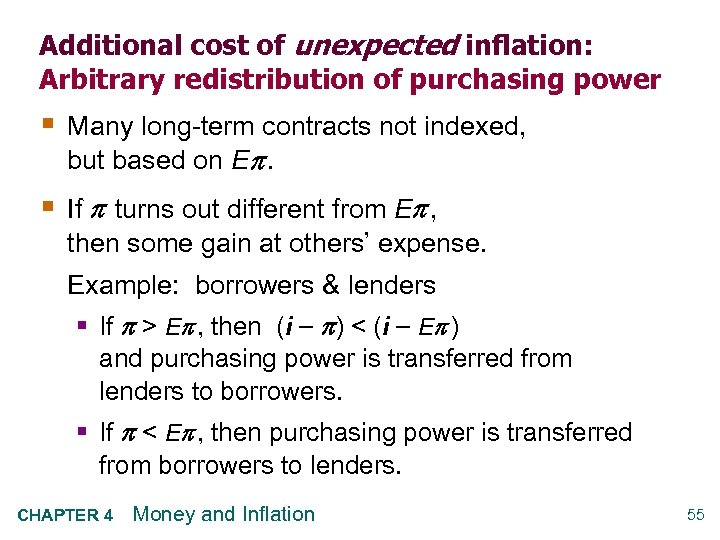Additional cost of unexpected inflation: Arbitrary redistribution of purchasing power § Many long-term contracts not indexed, but based on E . § If turns out different from E , then some gain at others’ expense. Example: borrowers & lenders § If > E , then (i ) < (i E ) and purchasing power is transferred from lenders to borrowers. § If < E , then purchasing power is transferred from borrowers to lenders. CHAPTER 4 Money and Inflation 55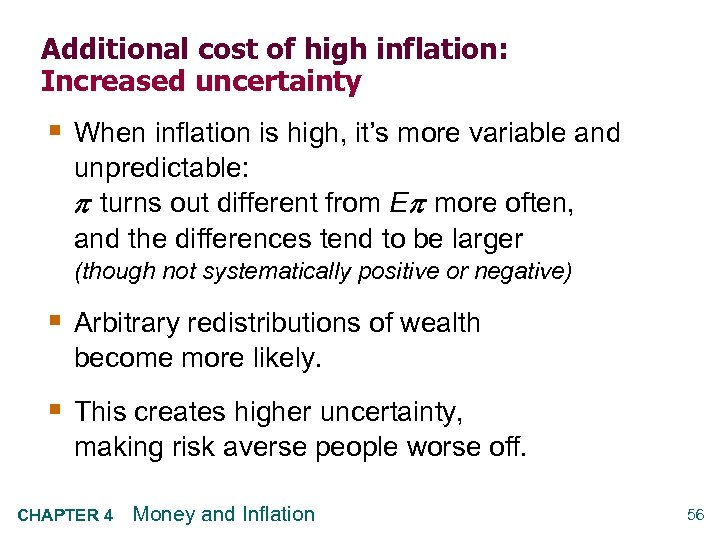Additional cost of high inflation: Increased uncertainty § When inflation is high, it’s more variable and unpredictable: turns out different from E more often, and the differences tend to be larger (though not systematically positive or negative) § Arbitrary redistributions of wealth become more likely. § This creates higher uncertainty, making risk averse people worse off. CHAPTER 4 Money and Inflation 56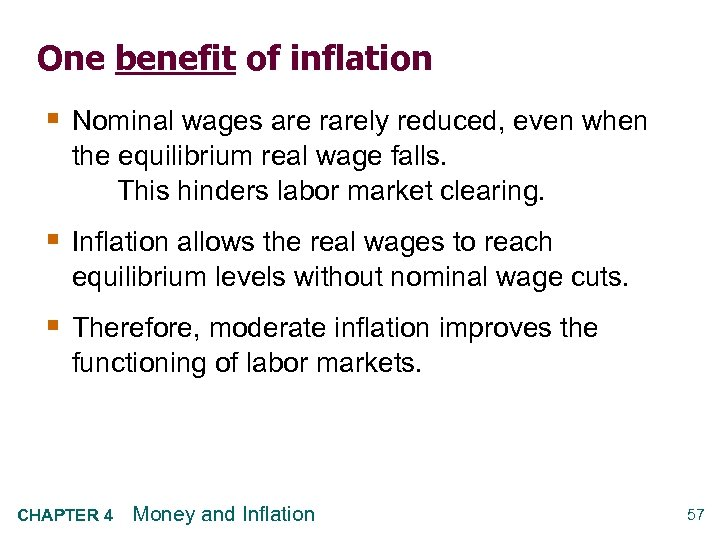One benefit of inflation § Nominal wages are rarely reduced, even when the equilibrium real wage falls. This hinders labor market clearing. § Inflation allows the real wages to reach equilibrium levels without nominal wage cuts. § Therefore, moderate inflation improves the functioning of labor markets. CHAPTER 4 Money and Inflation 57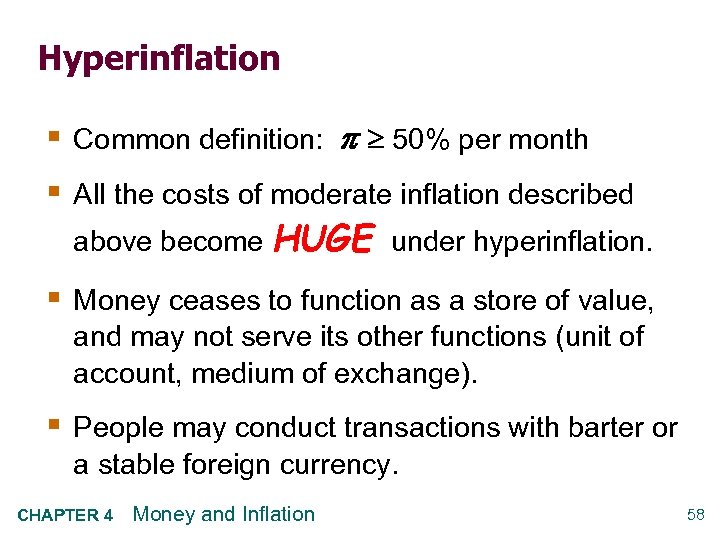Hyperinflation § Common definition: 50% per month § All the costs of moderate inflation described above become HUGE under hyperinflation. § Money ceases to function as a store of value, and may not serve its other functions (unit of account, medium of exchange). § People may conduct transactions with barter or a stable foreign currency. CHAPTER 4 Money and Inflation 58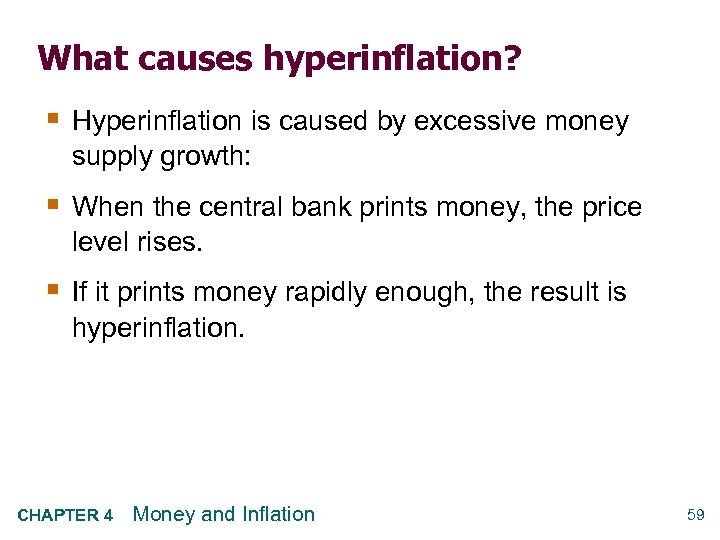What causes hyperinflation? § Hyperinflation is caused by excessive money supply growth: § When the central bank prints money, the price level rises. § If it prints money rapidly enough, the result is hyperinflation. CHAPTER 4 Money and Inflation 59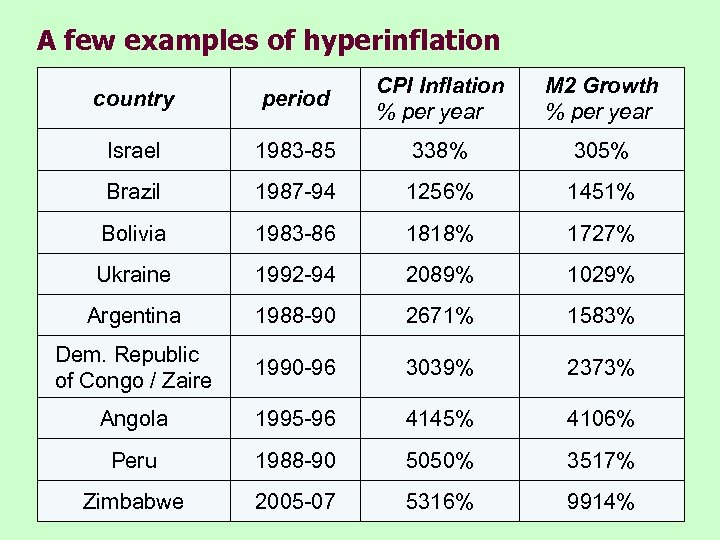A few examples of hyperinflation country period CPI Inflation % per year M 2 Growth % per year Israel 1983 -85 338% 305% Brazil 1987 -94 1256% 1451% Bolivia 1983 -86 1818% 1727% Ukraine 1992 -94 2089% 1029% Argentina 1988 -90 2671% 1583% Dem. Republic of Congo / Zaire 1990 -96 3039% 2373% Angola 1995 -96 4145% 4106% Peru 1988 -90 5050% 3517% Zimbabwe 2005 -07 5316% 9914%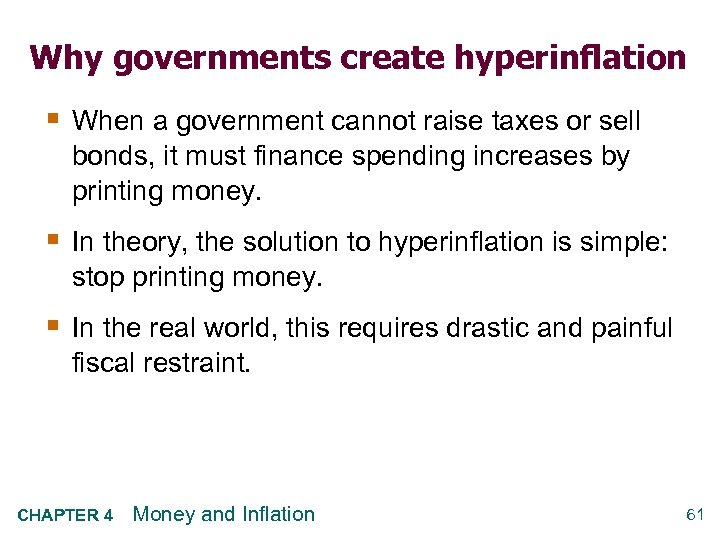Why governments create hyperinflation § When a government cannot raise taxes or sell bonds, it must finance spending increases by printing money. § In theory, the solution to hyperinflation is simple: stop printing money. § In the real world, this requires drastic and painful fiscal restraint. CHAPTER 4 Money and Inflation 61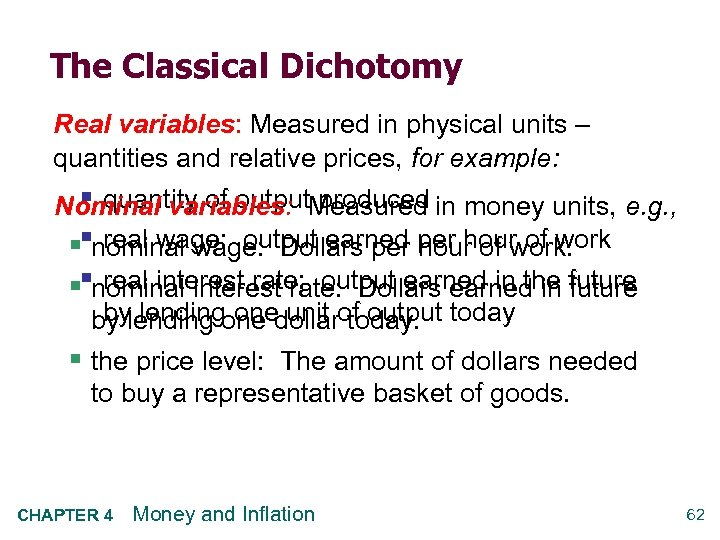The Classical Dichotomy Real variables: Measured in physical units – quantities and relative prices, for example: § quantity of output produced Nominal variables: Measured in money units, e. g. , real wage: output earned hour of work. §§nominal wage: Dollars per hour of work real interest rate: output earned in the future §§nominal interest rate: Dollars earned in future by lending one unit today. by lending one dollarof output today § the price level: The amount of dollars needed to buy a representative basket of goods. CHAPTER 4 Money and Inflation 62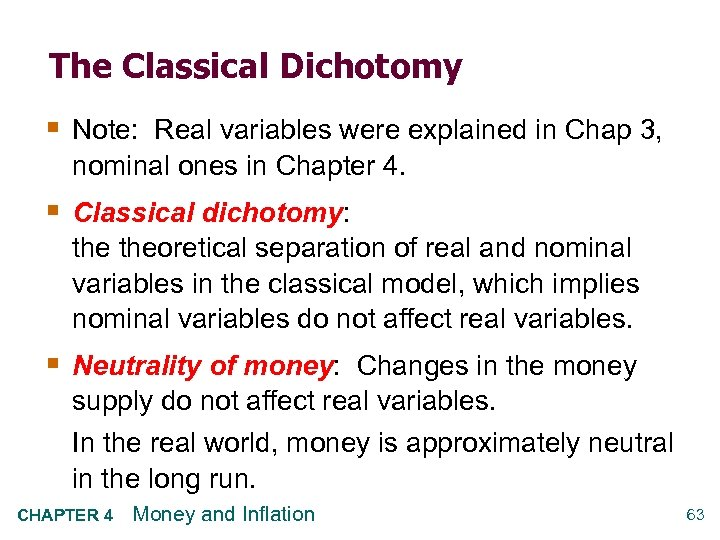The Classical Dichotomy § Note: Real variables were explained in Chap 3, nominal ones in Chapter 4. § Classical dichotomy: theoretical separation of real and nominal variables in the classical model, which implies nominal variables do not affect real variables. § Neutrality of money: Changes in the money supply do not affect real variables. In the real world, money is approximately neutral in the long run. CHAPTER 4 Money and Inflation 63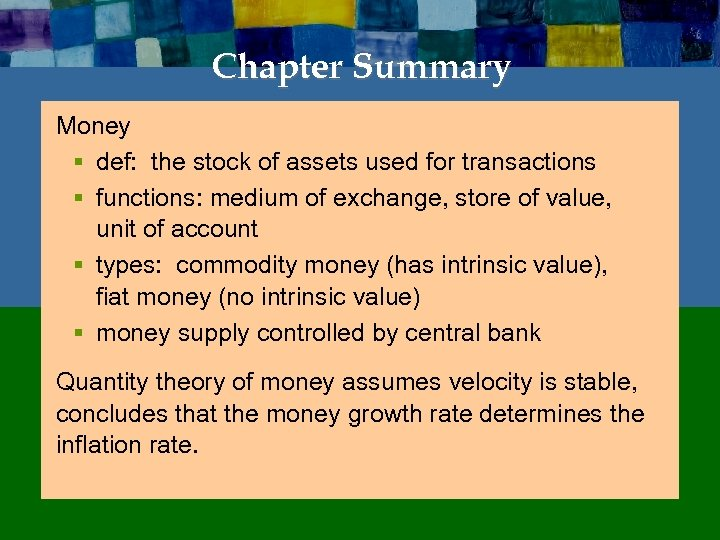Chapter Summary Money § def: the stock of assets used for transactions § functions: medium of exchange, store of value, unit of account § types: commodity money (has intrinsic value), fiat money (no intrinsic value) § money supply controlled by central bank Quantity theory of money assumes velocity is stable, concludes that the money growth rate determines the inflation rate.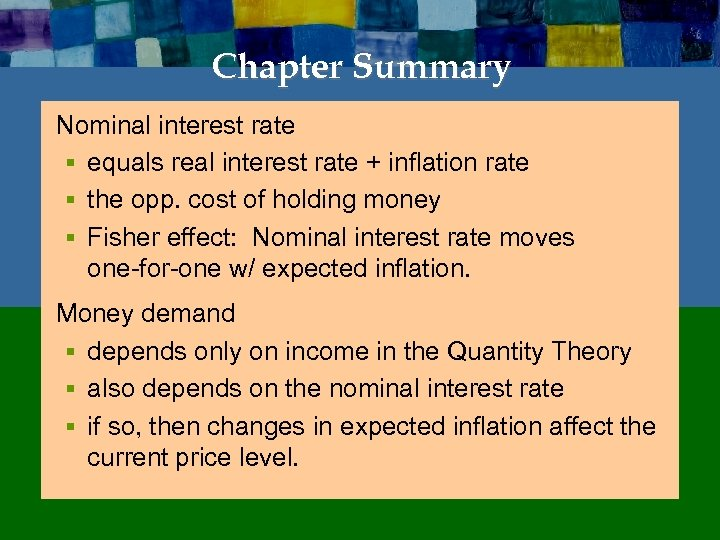Chapter Summary Nominal interest rate § equals real interest rate + inflation rate § the opp. cost of holding money § Fisher effect: Nominal interest rate moves one-for-one w/ expected inflation. Money demand § depends only on income in the Quantity Theory § also depends on the nominal interest rate § if so, then changes in expected inflation affect the current price level.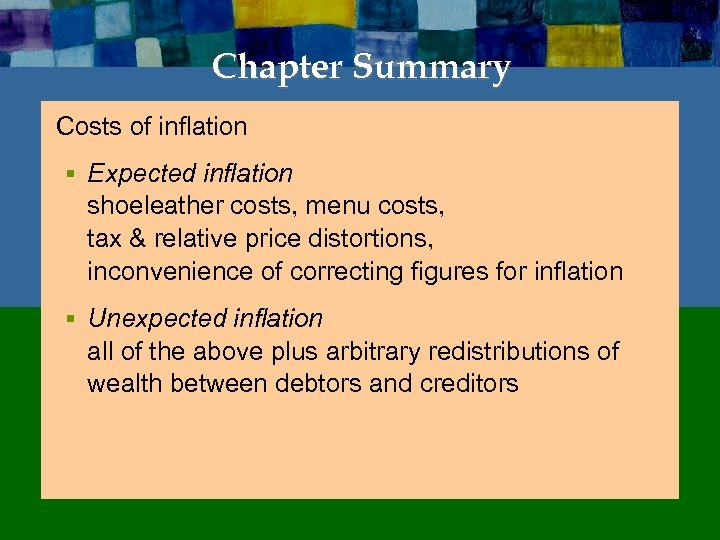Chapter Summary Costs of inflation § Expected inflation shoeleather costs, menu costs, tax & relative price distortions, inconvenience of correcting figures for inflation § Unexpected inflation all of the above plus arbitrary redistributions of wealth between debtors and creditors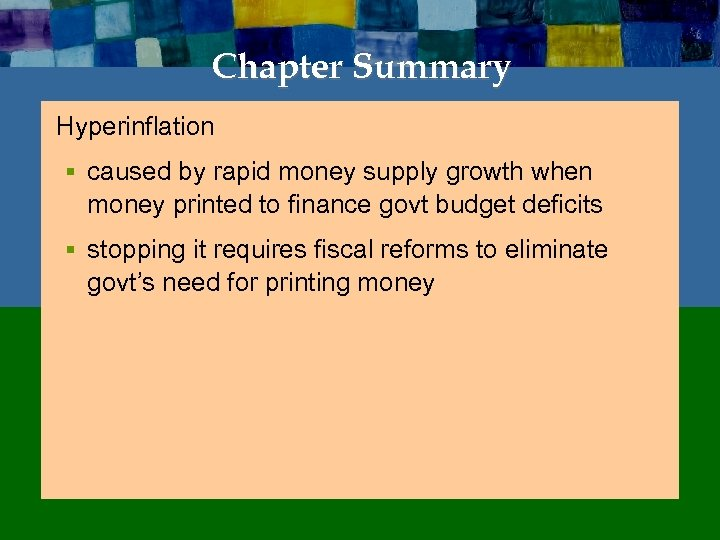Chapter Summary Hyperinflation § caused by rapid money supply growth when money printed to finance govt budget deficits § stopping it requires fiscal reforms to eliminate govt’s need for printing money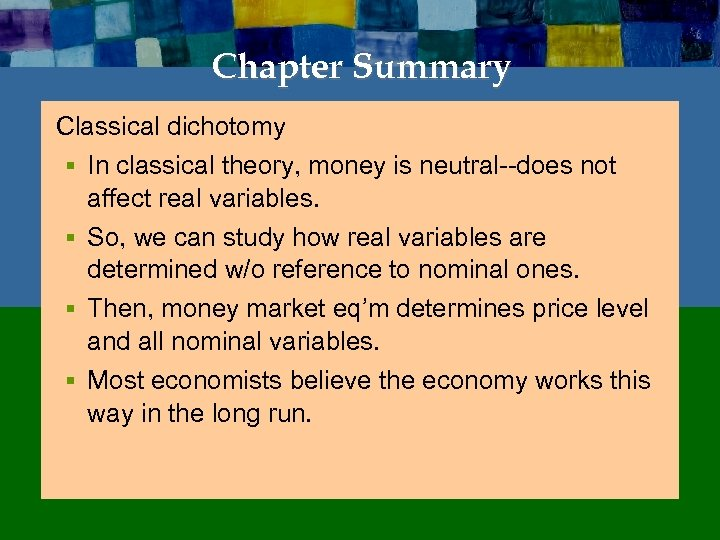Chapter Summary Classical dichotomy § In classical theory, money is neutral--does not affect real variables. § So, we can study how real variables are determined w/o reference to nominal ones. § Then, money market eq’m determines price level and all nominal variables. § Most economists believe the economy works this way in the long run.